×

Search anything:

# Max Pool and Avg Pool in TensorFlow

#### TensorFlow Machine Learning (ML)In this article, we have explored Max Pool and Avg Pool in TensorFlow in depth with Python code using the MaxPool and AvgPool ops in TensorFlow.

1. Introduction to Max Pool and Avg Pool
2. Max Pool in TF
3. Average Pooling in TF
4. Conclusion

## Introduction to Max Pool and Avg Pool

The convolutional neural network is made up of three layers namely: the convolution layer , the fully connected layer and the pooling layer. In this article we will take a look at the pooling layers , more specifically the max pooling and the average pooling layer.

What is the use of pooling layers?

Pooling layers are generally used to reduce the spatial size of the input representation given which infact reduces the parameters as well as lowers the computation time.The pooling layer helps in generalizing the features extracted from the convoltion layer.Generally the pooling layer helps in downsampling of features and hence is placed after the convolution layer.

How does the pooling layer function?
The pooling layer makes use of a mask/filter of smaller size than the input representation.This filter iterates over the convolved layer with a stride and performs a specific function.The specific function performed by the pooling layer varies depending on the type of pooling layer. There are different types of pooling layers, but the most widely used pooling layers are max pool , min pool and average pool.

The output generated from pooling has dimensions as below:

Height = (Input Height + Padding height (top and bottom) - Kernel Height) / Stride Height + 1
Width = (Input Width + Padding width (left and right) - Kernel Width) / Stride Width + 1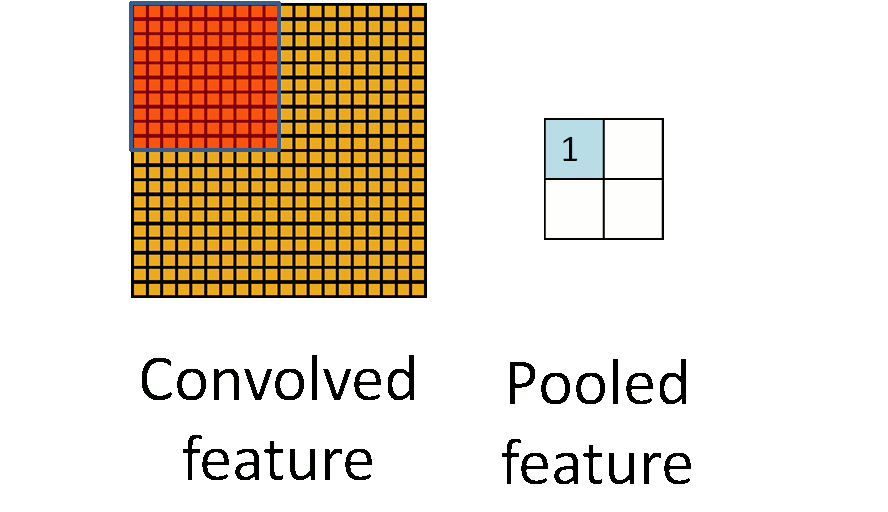## Max Pool in TF

When a filter of size k iterates over the convolved layer, it takes the maximum value present in each window. This window is of the same size as the filter. Max pool is quite helpful when feature extraction is required, as it only preserves information that is dominant and eliminates the rest.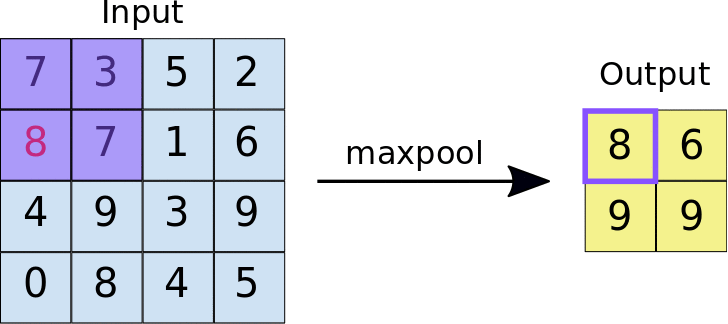Max pooling acts as a high pass filter meaning only higher range inputs will be able to pass through. It also reduces the size and makes the output concise.Maxpool has high translation invariance.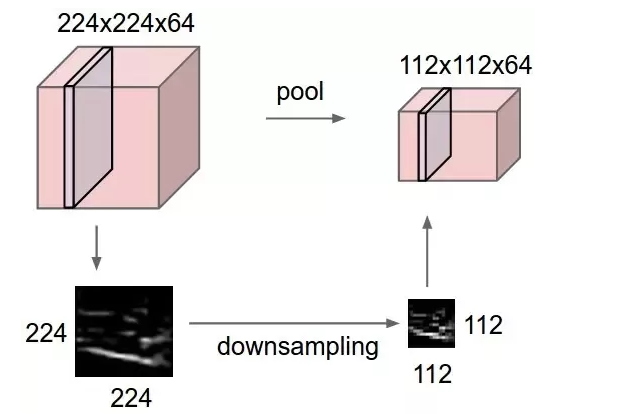Implementation:
The implementation of MaxPool is quite simple. The function tf.nn.max_pool() is used for max pooling.

Syntax:

``````tf.nn.max_pool(
input, ksize, strides, padding, data_format=None, name=None
)
``````

The max pool function has 4 essential parameters:

• input - for the input tensor
• ksize - for the size of the kernel/mask/filter
• strides - for the jump of each filter after every iteration
• padding - for the padding algorithm - either same or valid

Maxpool implementation:

``````matrix = tf.constant([
[1, 8, 2, 5],
[6, 7, 8, 9],
[0, 1, 2, 3],
[3, 2, 1, 1],
])
reshaped = tf.reshape(matrix, (1,4,4,1))
``````

maxpool() is an API which is exported from the below function:

``````def max_pool_v2(input, ksize, strides, padding, data_format=None, name=None):

if input.shape is not None:
n = len(input.shape) - 2
elif data_format is not None:
n = len(data_format) - 2
else:
raise ValueError(
"`input` must have a static shape or a data format must be given. "
f"data_format={data_format}")
if not 1 <= n <= 3:
raise ValueError(
f"`input.shape.rank` must be 3, 4 or 5. Received: "
f"input.shape={input.shape} of rank {n + 2}.")
if data_format is None:
channel_index = n + 1
else:
channel_index = 1 if data_format.startswith("NC") else n + 1

if isinstance(padding, (list, tuple)) and data_format == "NCHW_VECT_C":
raise ValueError("`data_format='NCHW_VECT_C'` is not supported with "

ksize = _get_sequence(ksize, n, channel_index, "ksize")
strides = _get_sequence(strides, n, channel_index, "strides")

if (isinstance(padding, (list, tuple)) and n == 3):
raise ValueError("Explicit padding is not supported with an input "

max_pooling_ops = {
1: max_pool1d,
2: max_pool2d,
3: gen_nn_ops.max_pool3d
}

op = max_pooling_ops[n]
return op(
input,
ksize=ksize,
strides=strides,
data_format=data_format,
name=name)
``````

Output:

``````<tf.Tensor: shape=(1, 2, 2, 1), dtype=int32, numpy=
array([[[,
],
[,
]]], dtype=int32)>
``````

## Average Pooling in TF

The working of the average pooling is very similar to that of the max pooling except the fact that the average is being taken into consideration of each patch that iterates over the feature map. The average of these patches is usually rounded of to the nearest decimal.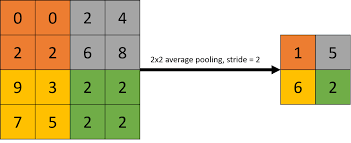A lot more data is lost when this pool is compared to that of max pool , since max pool focuses on the higher feature range, meaning high level features are preserved, meanwhile one low level feature could distort high level patches in average pooling technique.

Implementation:
The implementation of average pooling is very similar to max pooling:

``````tf.nn.avg_pool2d(
input, ksize, strides, padding, data_format='NHWC', name=None
)
``````

The above is an API , whereas the below code is

``````def avg_pool2d(input, ksize, strides, padding, data_format="NHWC", name=None):

with ops.name_scope(name, "AvgPool2D", [input]) as name:
if data_format is None:
data_format = "NHWC"
channel_index = 1 if data_format.startswith("NC") else 3

ksize = _get_sequence(ksize, 2, channel_index, "ksize")
strides = _get_sequence(strides, 2, channel_index, "strides")

return gen_nn_ops.avg_pool(
input,
ksize=ksize,
strides=strides,
data_format=data_format,
name=name)
``````

The difference between the two pooling layers i.e Max pooling layers and avg pooling can be seen in the image transformation below: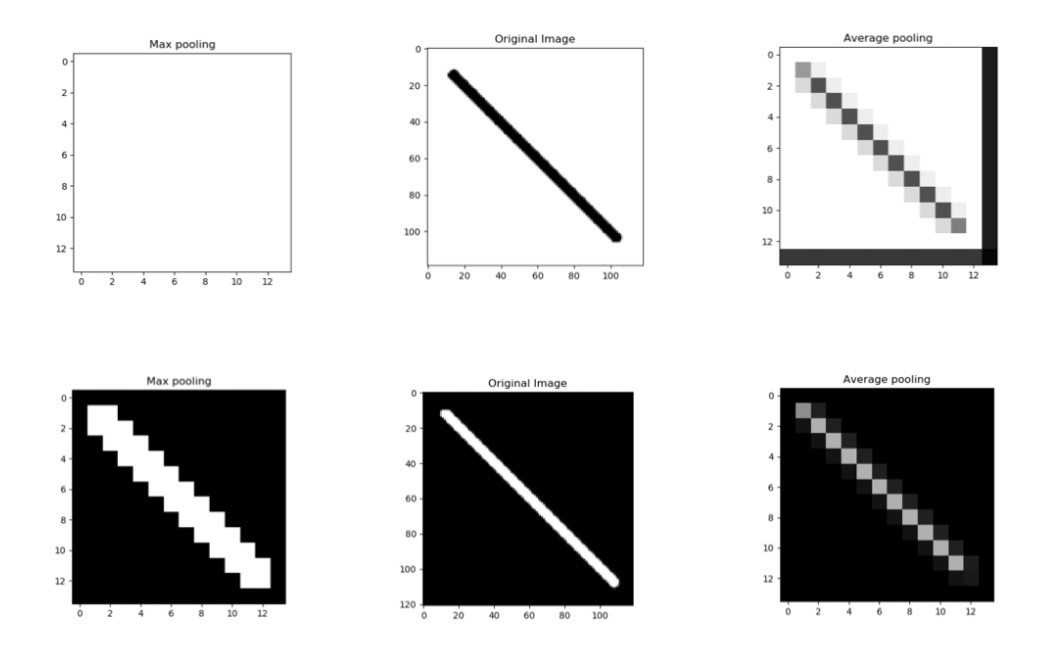## Conclusion

The pooling layers can be highly useful in cases where feature extraction,segmentation,enhancement and transformation are required. Also the data efficiency as well as the computational efficiency is highly improved with the help of these layers.